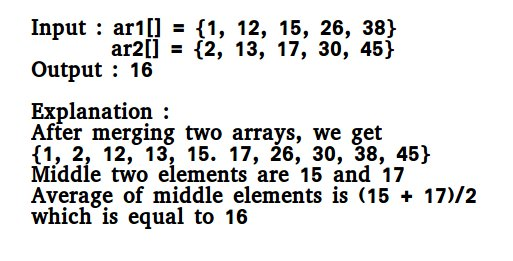# Java Program for Median of two sorted arrays of same size

• Last Updated : 11 Dec, 2021

There are 2 sorted arrays A and B of size n each. Write an algorithm to find the median of the array obtained merging the above 2 arrays(i.e. array of length 2n). The complexity should be O(log(n)).## Recommended: Please solve it on “PRACTICE ” first, before moving on to the solution.

Note : Since size of the set for which we are looking for median is even (2n), we need take average of middle two numbers and return floor of the average.

Method 1 (Simply count while Merging)
Use merge procedure of merge sort. Keep track of count while comparing elements of two arrays. If count becomes n(For 2n elements), we have reached the median. Take the average of the elements at indexes n-1 and n in the merged array. See the below implementation.

## Java

 `// A Simple Merge based O(n) solution``// to find median of two sorted arrays`` ` `class` `Main {``    ``// function to calculate median``    ``static` `int` `getMedian(``int` `ar1[], ``int` `ar2[], ``int` `n)``    ``{``        ``int` `i = ``0``;``        ``int` `j = ``0``;``        ``int` `count;``        ``int` `m1 = -``1``, m2 = -``1``;`` ` `        ``/* Since there are 2n elements, median will ``           ``be average of elements at index n-1 and ``           ``n in the array obtained after merging ar1 ``           ``and ar2 */``        ``for` `(count = ``0``; count <= n; count++) {``            ``/* Below is to handle case where all ``              ``elements of ar1[] are smaller than ``              ``smallest(or first) element of ar2[] */``            ``if` `(i == n) {``                ``m1 = m2;``                ``m2 = ar2[``0``];``                ``break``;``            ``}`` ` `            ``/* Below is to handle case where all ``               ``elements of ar2[] are smaller than ``               ``smallest(or first) element of ar1[] */``            ``else` `if` `(j == n) {``                ``m1 = m2;``                ``m2 = ar1[``0``];``                ``break``;``            ``}`` ` `            ``if` `(ar1[i] < ar2[j]) {``                ``/* Store the prev median */``                ``m1 = m2;``                ``m2 = ar1[i];``                ``i++;``            ``}``            ``else` `{``                ``/* Store the prev median */``                ``m1 = m2;``                ``m2 = ar2[j];``                ``j++;``            ``}``        ``}`` ` `        ``return` `(m1 + m2) / ``2``;``    ``}`` ` `    ``/* Driver program to test above function */``    ``public` `static` `void` `main(String[] args)``    ``{``        ``int` `ar1[] = { ``1``, ``12``, ``15``, ``26``, ``38` `};``        ``int` `ar2[] = { ``2``, ``13``, ``17``, ``30``, ``45` `};`` ` `        ``int` `n1 = ar1.length;``        ``int` `n2 = ar2.length;``        ``if` `(n1 == n2)``            ``System.out.println(``"Median is "` `+ getMedian(ar1, ar2, n1));``        ``else``            ``System.out.println(``"arrays are of unequal size"``);``    ``}``}`

Method 2 (By comparing the medians of two arrays)

This method works by first getting medians of the two sorted arrays and then comparing them.

## Java

 `// A Java program to divide and conquer based``// efficient solution to find``// median of two sorted arrays``// of same size.``import` `java.util.*;``class` `GfG {`` ` `    ``/* This function returns median ``of ar1[] and ar2[]. ``Assumptions in this function: ``    ``Both ar1[] and ar2[] are ``    ``sorted arrays ``    ``Both have n elements */``    ``static` `int` `getMedian(``int` `ar1[], ``int` `ar2[], ``int` `n)``    ``{``        ``/* return -1 for ``    ``invalid input */``        ``if` `(n <= ``0``)``            ``return` `-``1``;``        ``if` `(n == ``1``)``            ``return` `(ar1[``0``] + ar2[``0``]) / ``2``;``        ``if` `(n == ``2``)``            ``return` `(Math.max(ar1[``0``], ar2[``0``]) + Math.min(ar1[``1``], ar2[``1``])) / ``2``;`` ` `        ``/* get the median of ``    ``the first array */``        ``int` `m1 = median(ar1, n);`` ` `        ``/* get the median of ``    ``the second array */``        ``int` `m2 = median(ar2, n);`` ` `        ``/* If medians are equal then ``    ``return either m1 or m2 */``        ``if` `(m1 == m2)``            ``return` `m1;`` ` `        ``/* if m1 < m2 then median must ``    ``exist in ar1[m1....] and ``                ``ar2[....m2] */``        ``if` `(m1 < m2) {``            ``if` `(n % ``2` `== ``0``)``                ``return` `getMedian(ar1 + n / ``2` `- ``1``, ar2, n - n / ``2` `+ ``1``);``            ``return` `getMedian(ar1 + n / ``2``, ar2, n - n / ``2``);``        ``}`` ` `        ``/* if m1 > m2 then median must ``    ``exist in ar1[....m1] and ``                ``ar2[m2...] */``        ``if` `(n % ``2` `== ``0``)``            ``return` `getMedian(ar2 + n / ``2` `- ``1``, ar1, n - n / ``2` `+ ``1``);``        ``return` `getMedian(ar2 + n / ``2``, ar1, n - n / ``2``);``    ``}`` ` `    ``/* Function to get median ``of a sorted array */``    ``static` `int` `median(``int` `arr[], ``int` `n)``    ``{``        ``if` `(n % ``2` `== ``0``)``            ``return` `(arr[n / ``2``] + arr[n / ``2` `- ``1``]) / ``2``;``        ``else``            ``return` `arr[n / ``2``];``    ``}`` ` `    ``// Driver code``    ``public` `static` `void` `main(String[] args)``    ``{``        ``int` `ar1[] = { ``1``, ``2``, ``3``, ``6` `};``        ``int` `ar2[] = { ``4``, ``6``, ``8``, ``10` `};``        ``int` `n1 = ar1.length;``        ``int` `n2 = ar2.length;``        ``if` `(n1 == n2)``            ``System.out.println(``"Median is "` `+ getMedian(ar1, ar2, n1));``        ``else``            ``System.out.println(``"Doesn't work for arrays "``                               ``+ ``"of unequal size"``);``    ``}``}`

Please refer complete article on Median of two sorted arrays of same size for more details!

My Personal Notes arrow_drop_up